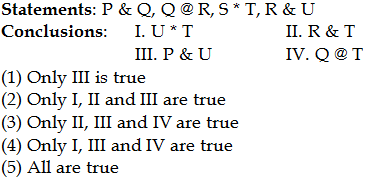## PREPARATORY QUESTIONS

### EQUAL-INEQUAL

#### PREPARATORY SET-72 (HARD)

Directions (Qs.1 to 5): Study the following information carefully to answer the given questions.

‘A % B’ means ‘A is neither smaller nor equal to B'

‘A & B’ means ‘A is neither greater nor equal to B’

‘A \$ B’ means ‘A is not smaller than B’

‘A * B’ means ‘A is neither smaller nor greater than B’

‘A @ B’ means ‘A is not greater than B’

Now in each of the following questions, assuming the given statements to be true, find which of the two conclusions given below them is/are true.

Question No : 1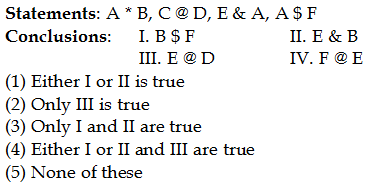Question No : 2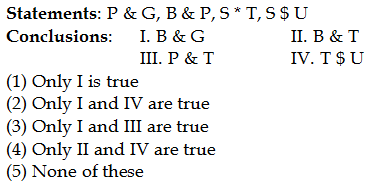Question No : 3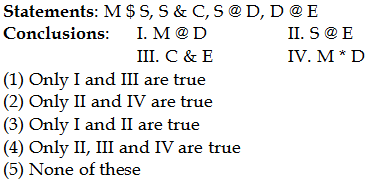Question No : 4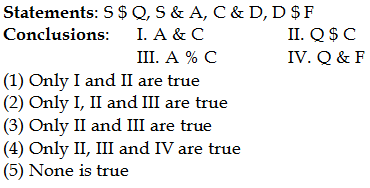Question No : 5